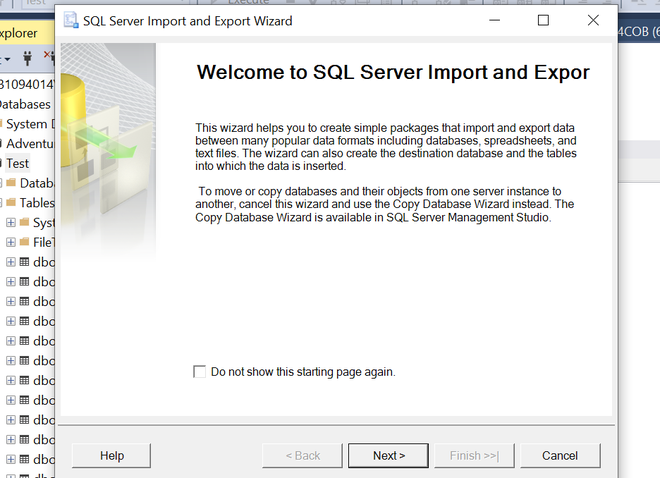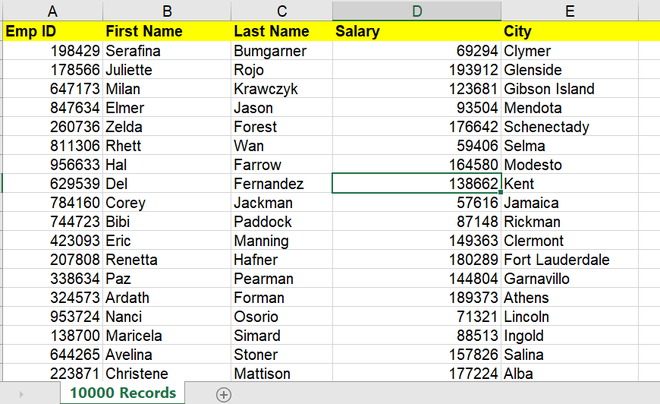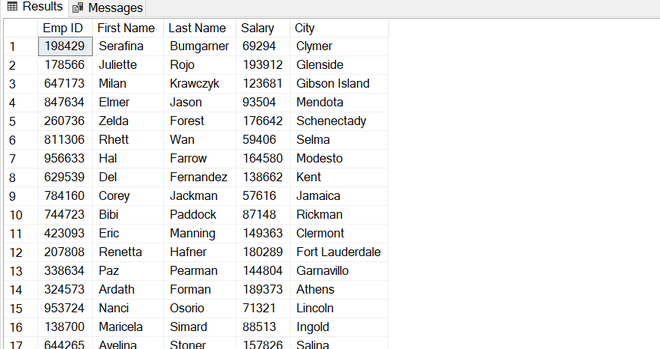Open in App
Not now

# SQL SERVER | Bulk insert data from csv file using T-SQL command

• Difficulty Level : Basic
• Last Updated : 09 Mar, 2021

In this article, we will cover bulk insert data from csv file using the T-SQL command in the SQL server. And will also cover the way is more useful and more convenient to perform such kind of operations. Let’s discuss it one by one.

Introduction :
Sometimes there is a scenario when we have to perform bulk insert data from .csv files into SQL Server database. We can use the GUI interface in SSMS(SQL Server Management Studio) to import data from Excel, CSV, etc files. What if we have millions of data to be imported, the above will be a time-consuming task so, now you will see how you can handle such kinds of operation.Note –
Requires INSERT and ADMINISTER BULK OPERATIONS permissions.

Alternative Approach :
Here, in this article, we will provide a faster alternative to the above via few lines of T-SQL Command.

```BULK INSERT <DATABASE NAME>.<SCHEMA NAME>.<TABLE_NAME>
FROM '<FILE_PATH>'
WITH
(
-- input file format options
[ [ , ] FORMAT = 'CSV' ]
[ [ , ] FIELDQUOTE = 'quote_characters']
[ [ , ] FORMATFILE = 'format_file_path' ]
[ [ , ] FIELDTERMINATOR = 'field_terminator' ]
[ [ , ] ROWTERMINATOR = 'row_terminator' ]
)]
)```

The most commonly used input parameters are the following as follows.

1. FIELDTERMINATOR –
‘field_terminator’ is used as a separator between fields. The default field terminator is \t (tab character).

2. ROWTERMINATOR –
‘row_terminator’ is used to specify the end of the row. The default row terminator is \r\n (newline character).

Importing CSV file into SQL table :
Here, we will discuss importing CSV file into a table in SQL server. Consider the given below CSV file or you can use your own csv file to perform this operation. Let us try to import a csv file into our SQL table in SQL Server.Now, let’s create the SQL table to store the above data.

Creating table –
Here. We will create the table schema as per csv file records.

```USE [Test]--Database name

CREATE TABLE [dbo].[Employee](
[Emp ID] bigint primary key,
[First Name] [varchar](50) NULL,
[Last Name] [varchar](50) NULL,
[Salary] bigint,
[City] [varchar](50)
)```

Bulk Inserting –
Let’s now bulk insert the file into the Employee SQL table :

```BULK INSERT [Test].[dbo].[Employee]
FROM 'C:\data\employee_data.csv'
WITH (FIRSTROW = 2,
FIELDTERMINATOR = ',',
ROWTERMINATOR='\n' );```

Output –
After executing the above operation we can see the records have been inserted with few lines of code into the Employee table like below.References :